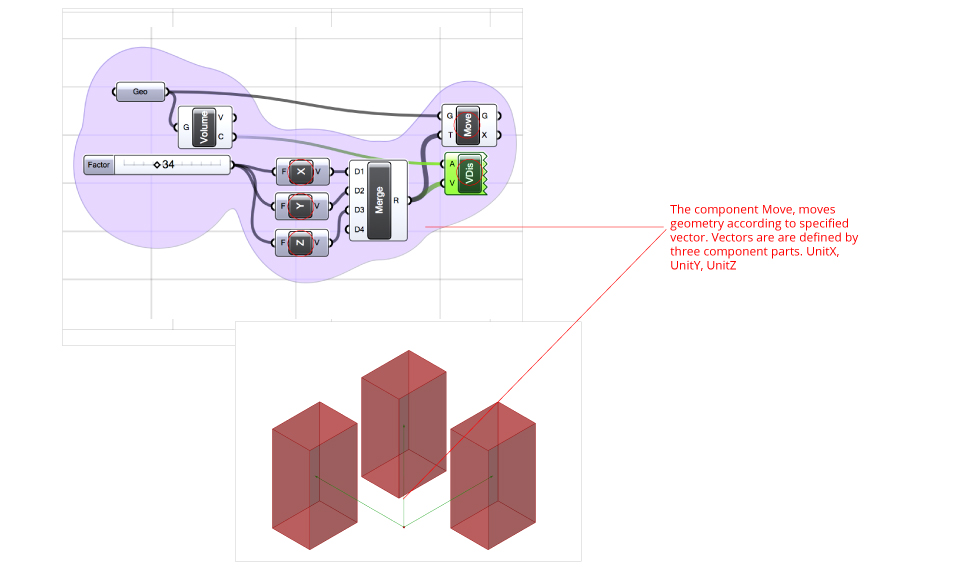Basic concepts and operations – Grasshopper

Object Snap – The object snap tool in in Rhino allows us to select a specific strategic location to connect to objects. i.e; mid point, end point, etc. Grasshopper does not work under this logic. Instead, it relies on algorithm logic to recreate this functionality. The Point on Curve component defines a point on a curve by parameterizing the curve’s length.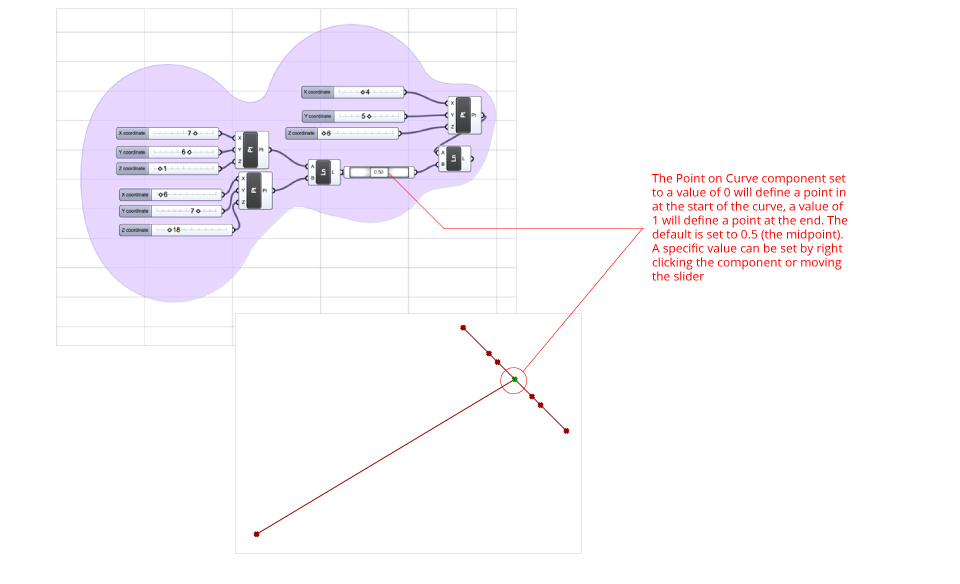End Points – The component End Points can be used to extract the end and start point of a given curve. In this example the component is used to access the end and start points of a curve to generate two lines between the start and end points and a third defined point.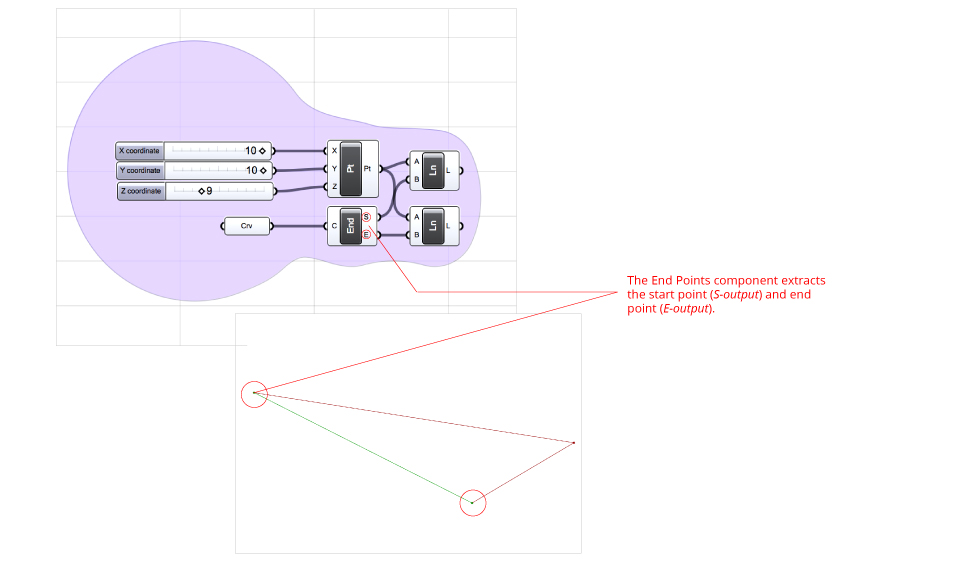Area – The component Area can be used to extract the geometry centroid of a planar curve. It also calculates the area. The example demonstrates how the Area component can be used to access the centroids of two closed curves, then connect the points to create a line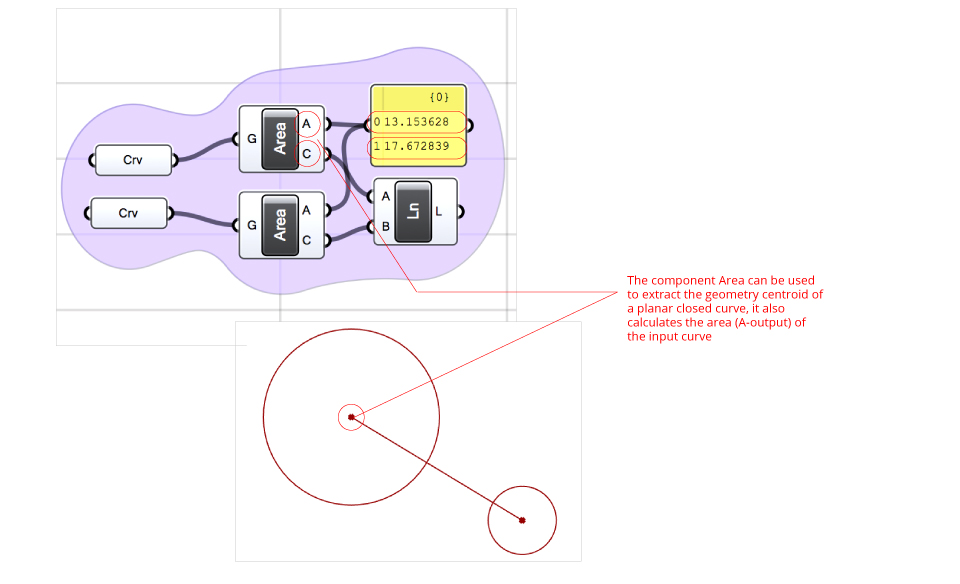Volume – The component Volume can be used to extract the geometry centroid of a closed three dimensional object (C-output). It also calculates the volume (V-output). The example demonstrates how the Volume component can be used to access the centroids of a closed curve and a three dimensional object then connect the points to create a lineMerge – The component Merge allows two or more data flows to converge in a single list. The example below shows how to use just one line component instead of two, by collecting the start point and end point by the merge component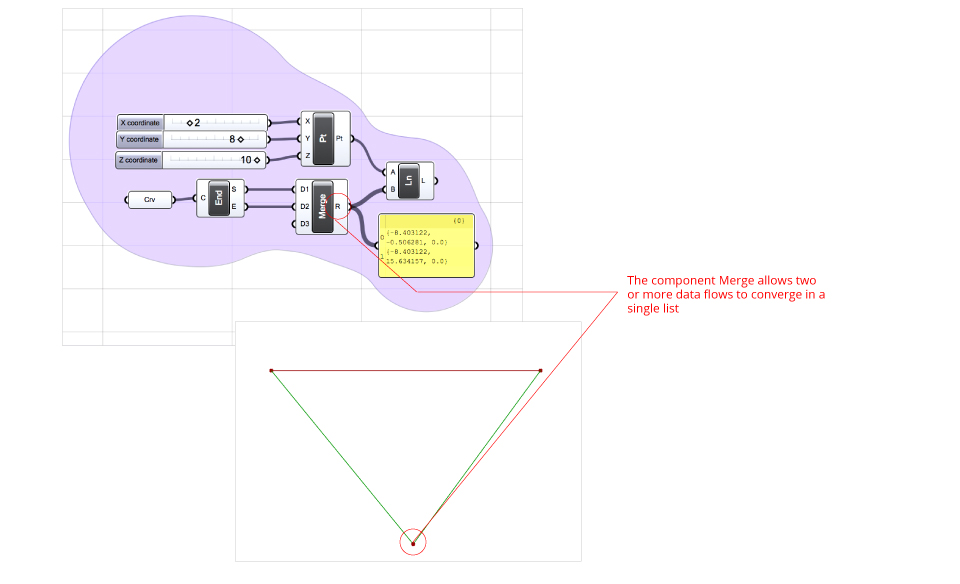Divide Curve – The component Divide Curve divides a closed or open curve into equal segments. Given a curve set from Rhino (C – input) and an integer for the number of subdivisions  (N-input), N points (P-output)  will be generated in case of an open curve and N+1 points in case of an open curve. Points are store in a single list. The index number of each point and the points order can be displayed using tge component Point. Display components do not have an output section as they are used for visualization. The component Point List allows users to set the text size by (S-input)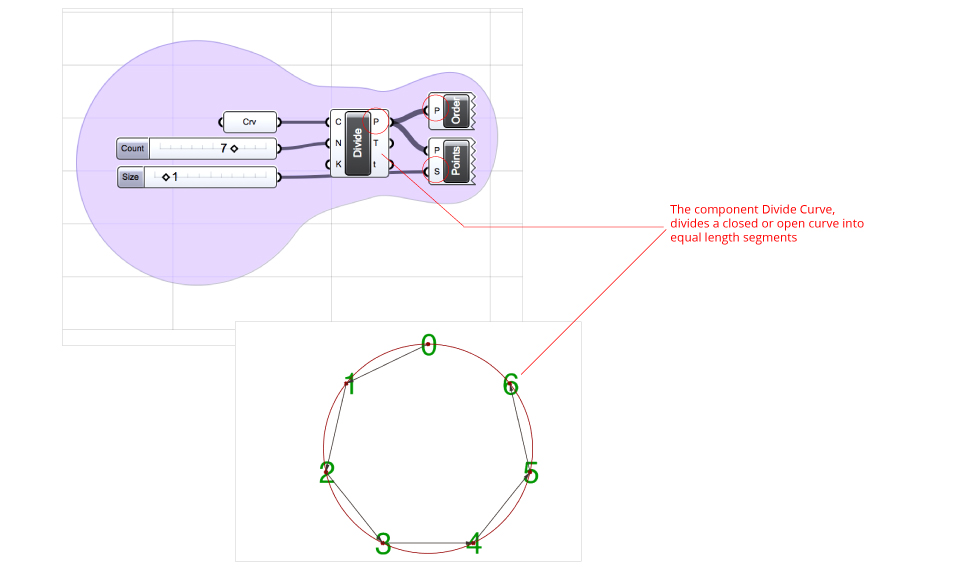Move – The component Move, moves geometries according to a specified vector. The following example uses the Move component to translate an input geometry (G) along a vector (T). The vector is defined through UnitX, UnitY and UnitZ whose direction is parallel and sense to its axis and length equal to 1. In order to amplify the vector and consequently the movement it is enough to connect a Number Slider To the F-input of UnitX, UnitY and UnitZ.